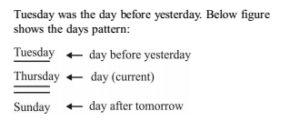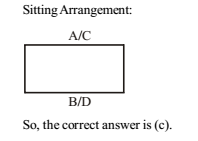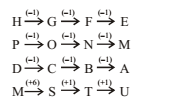# RRB ALP 2018 Practice Test Papers | Reasoning Questions (Day-44)

Dear Aspirants, Here we have given the Important RRB ALP & Technicians Exam 2018 Practice Test Papers. Candidates those who are preparing for RRB ALP 2018 can practice these Reasoning Questions to get more confidence to Crack RRB 2018 Examination.

[WpProQuiz 2229]

Click “Start Quiz” to attend these Questions and view Solutions

1. If the day to day-after-tomorrow is Sunday, which day was day-before-yesterday?

(a) Thursday

(b) Wednesday

(c) Tuesday

(d) Monday

1. The digits are given as below: 562, 871, 438, 753 If the position of the first and the third digits of each of the numbers are interchanged, which of the following will be the sum of the first and the second digits of the third highest number?

(a) 9

(b) 7

(c) 6

(d) 8

1. If 33 + 45 = 30, 90 + 26 = 40, then 30 + 45 = — ?

(a) 15

(b) 14

(c) 16

(d) 18

1. The average age of 25 suboridinates in an office is 30 years. If the age of Manager is added, the average increases to 31 years. What is the age of the Manager?

(a) 26

(b) 36

(c) 46

(d) 56

1. Four friends ABCD are sitting in a coffee shop. A and B are sitting face to face. D is not sitting next to A but can see the facial expression of A clearly. B is talking to C who is sitting opposite to him. Who are sitting together?

(a) D & C

(b) A & B

(c) A & C

(d) A & D

DIRECTIONS (Qs. 6 – 7): In questions, find the odd word/letters/ number pair from the given alternatives.

1. (a) Kolkata

(b) Vishakhapatnam

(c) Bengaluru

(d) Haldia

1. (a) HGFE

(b) PONM

(c) DCBA

(d) MSTU

DIRECTIONS (Q. 8): In questions below, a series is given with one term missing. Choose the correct alternative from the given ones that will complete the series.

1. 3, 6, 9, 15, 24, 39, 63,?

(a) 100

(b) 87

(c) 102

(d) 99

1. If A = l, AGE = 13, then CAR =?

(a) 19

(b) 20

(c) 21

(d) 22

1. After interchanging ¸ and =, 2 and 3 which one of the following statements becomes correct?

(a) 15 = 2 ÷ 3

(b) 5 ÷15 = 2

(c) 2 = 15 ÷ 3

(d) 3 = 2 ÷15

1. Answer: (c)Arranging digits according to question. 265, 178, 834, 357 Hence, third highest would be 265 and 8 be the sum of first and second digits of the number.

As, 3 + 3 + 4 + 5 = 15

=> 1 + 5 = 6 and, 6 × 5 = 30

9 + 0 + 2 + 6 = 17

=> 1 + 7 = 8 and, 8 × 5 = 40 Similarly, 3 + 0 + 4 + 5 = 12 => 1 + 2 = 3 and, 3 × 5 = 15

Age of Manager = New Average Age + (No. of Subordinates × Change in Average) = 31 + (25 × 1) = 56 years

1. Answer: (c)DIRECTIONS (Qs. 6 – 7):

2. Answer: (d)DIRECTIONS (Q. 8):

3 +3 = 6

6 + 3 = 9

9 + 6 = 15

15 + 9 = 24

24 + 15 = 39

39 + 24 = 63

63 + 39 = 102

As, A + G + E = 1 + 7 + 5 = 13 Similarly, C + A + R = 3 + 1 + 18 = 22

5 = 15 ÷ 3

RRB ALP 2018 – “All in One” Study Materials and Practice Sets